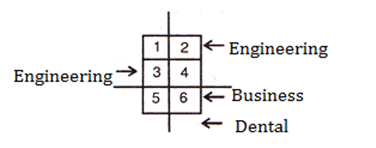### SBI PO high level reasoning questions: SBI PO high level reasoning questions that you should solve to target a high score

To target a high score in the reasoning section of the SBI PO and other competitive exams, solve the SBI PO high level reasoning questions. If you have started with Logical Reasoning sets provided here, continue solving all the Level-3 sets to get a flavour of the difficult problems that could be asked in the exams. SBI PO high level reasoning questions cover the most essential topics that are asked in the Reasoning section of most of the competitive exams and are great performance boosters. Before you start solving the Level-3 problem sets, thoroughly go through the concepts of each topic individually in order to gain maximum score and confidence through this exercise.

Directions for the question set:
Six square states having equal area in a country are located in North-South directions in two columns next to each other. States are located in the given order, State 1, State 3, and State 5 are on the western side and State 2, State 4, and State 6 are on the eastern side. Within the six states, there are exactly four Dental institutes, two business institutes, and two Engineering institutes. These eight institutions are located as follows:
No institution is in more than one of the states.
None of the states contains more than one business institute, and none contains more than one Engineering institute.
None of the states contains both a business institute and an Engineering institute.
Each business institute is located in a state that contains at least one Dental institute.
The Engineering institutes are located in two states that do not share a common boundary.
State 3 contains an Engineering institute, and State 6 contains a business institute.

Question 1: If one of the states contains exactly two Dental institutes and exactly one Engineering institute, then which combination of three states might contain no Dental institute?
A. 1, 4, 5
B. 2, 3, 5
C. 2, 4, 6
D. 1,3, 5
E. 4, 5, 6

Question 2. Which one of the following could be true?
A. State 1 contains exactly one Engineering institute
B. State 2 contains exactly one business institute
C. State 5 contains exactly one Engineering institute
D. State 6 contains exactly one Engineering institute
E. State 1 contains exactly one Dental institute

Question 3: A complete and accurate list of the states, any one of which could contain the business Institute that is not in State 6, would be________.
A. 1, 4
B. 2, 4
C. 1, 4, 5
D. 4, 5
E. 1, 2, 4, 5

Question 4: If each of the six states contains at least one of the eight institutions, then which one of the following must be true?
A. There is business institute in state 1
B. There is a Dental institute in state 2
C. There is a Dental institute in state 3
D. There is a Dental institute in state 4
E. There is a business institute in state 4

### Answers and Explanations: Click the down arrow to expand

Common solution for the set: State 3 contains Engineering institute and state 6 contains a business institute. State 6 cannot contain an Engineering institute and also states 1, 4 and 5 cannot contain an Engineering institute as they share the common boundary. Therefore, state 2 contains an Engineering institute. From the given information and above conclusions the following diagram can be drawn.Answer 1: (D) Because dental institute can only be in 2, 4 and 6 states as per the condition, it cannot be in 1,3,5. The correct option is (D).

Answer 2: (E) Engineering institutes are already assigned to state 2 and 3. So option A,C and D can be eliminated. 2 cannot be assigned business institute as per condition. The correct option is (E).

Answer 3: (C) 2 and 3 states cannot have business institute as per condition. So, option B and E can be eliminated. Rest state can be assigned Business institute. The correct option is (C).

Answer 4: (D) For the common solution for this set, refer to solution of Question 1. According to the condition, there is a Dental institute in state 4 The correct option is (D).

Extra tips for SBI PO high level reasoning questions:
• While solving SBI PO high level reasoning questions in the mock and main exam, use tables and figures to derive the solution as it becomes easier to visualize the problem and reach to the answer.
• Make notes while you learn theory and refer them every time you take a test.
• Use option elimination while solving SBI PO high level reasoning questions to save time in the exam.